Home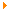Teaching AeraMy Teaching
 My Teaching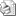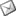Teaching
Written by Romain Raveaux
Mar 05, 2009 at 10:49 AM
Here is a description of the subjects I have taught so far. Teaching material I have designed myself can be downloaded and re-used freely.

I am a teacher at Polytech’Tours engineering school of the university of Tours.
• Networking: 127 h per year (Level L3) from 2013 to 2019.
• Mobile programming: 36 h per year (Level M2) from 2013 to 2019.
• Multimedia systems: 18 h per year (Level M2) from 2013 to 2019.
• Python and data science: 38 h per year (Level L2) from 2016 to 2019.
• System programming: 12 h per year (Level L3) shell, fork, shared memory, pthread, semaphore. From 2012 to 2014.
• Pattern recognition: 8h per year (Level M2) from 2015 to 2017.
• Industrial Networking: 12h per year. CAN protocol (Level M1) from 2015 to 2017.
• C for microcontroller: 10h per year (Level M1) from 2015 to 2017.

### Graph Neural Network: a course and a notebook

Goals :
• An illustration of Graph Neural Networks for graph classification
• A toy application to Letter classification
• A simple Graph Neural Network from Scratch (only with PyTorch and Networkx)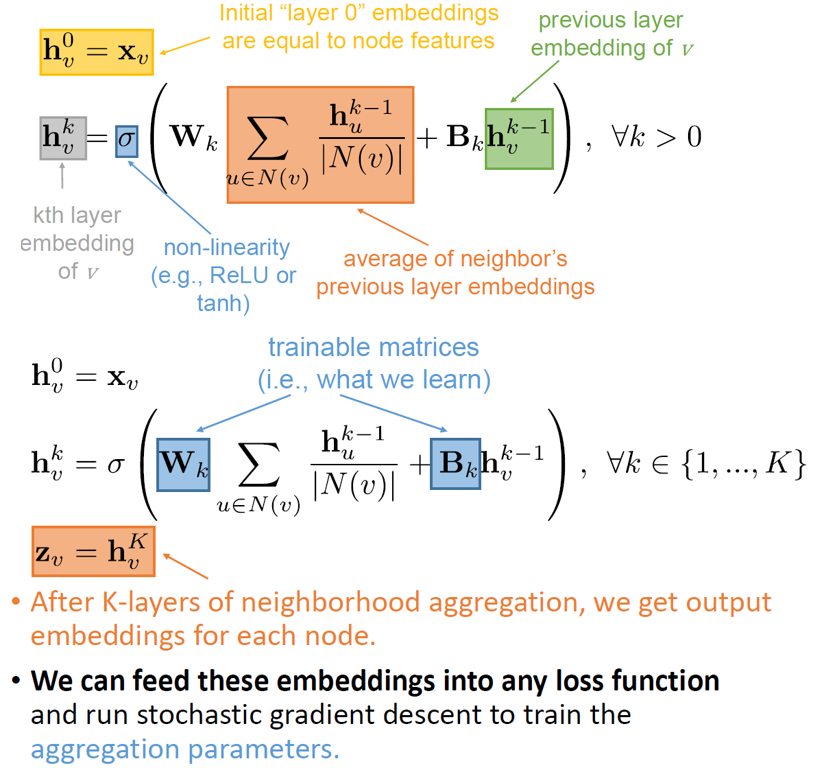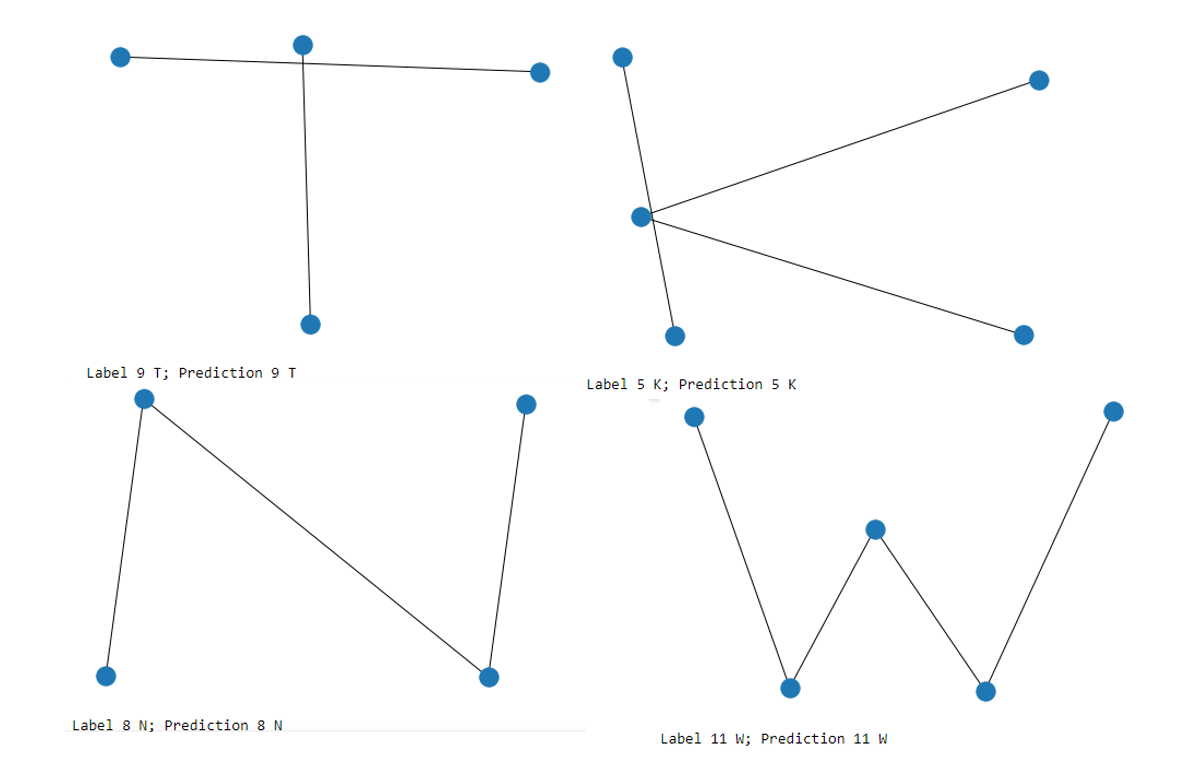• Introduction to supervised Machine Learning: A probabilistic introduction. PDF slides
• Connecting local models : The case of chains PDF slides
• Graph Neural Networks. PDF slides
• The tutorial can be found here. HTML Notebook

A follow-up tutorial entitled "A Graph Neural Network from Scratch for semantic image segmentation".
Goals :
• An illustration of Graph Neural Network for node classification
• A toy application to skin detection
• A simple Graph Neural Network from Scratch (only with PyTorch and Networkx)
• The tutorial can be found here. HTML Notebook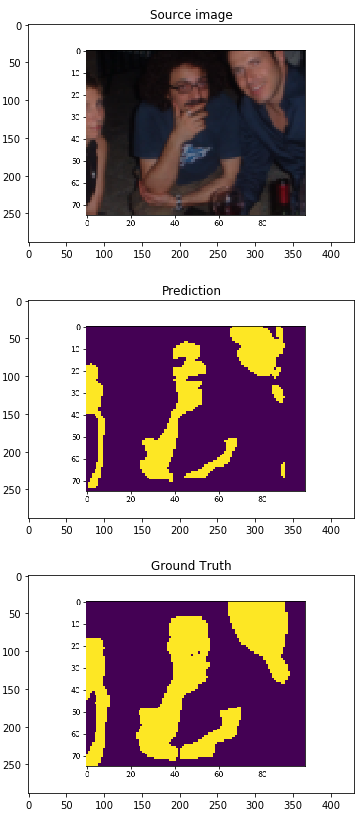A follow-up tutorial entitled "A Graph Neural Network (GNN) from Scratch for semi-supervised learning".
Goals :
• An illustration of GNN for semi-supervised learning
• A toy applicaion to community detection
• A lecture on Structured Machine Learning
• A simple Graph Neural Network from Scratch (only with PyTorch and Networkx)
• The tutorial can be found here. HTML Notebook
Supervised classification : Unsupervised classification : Semi-supervised classification :

### Markov Random Field : A simple application to de-noising image

The application of undirected graphs using an example of noise removal from a binary image and gray image. Goals :
• An introduction to probabilistic graphical models.
• An application to Image Denoising.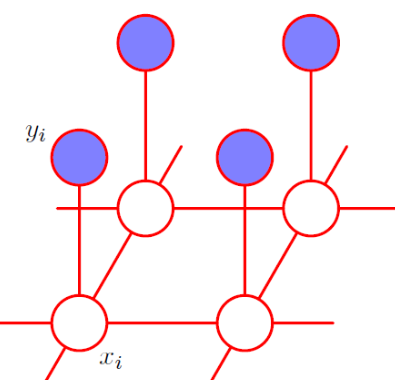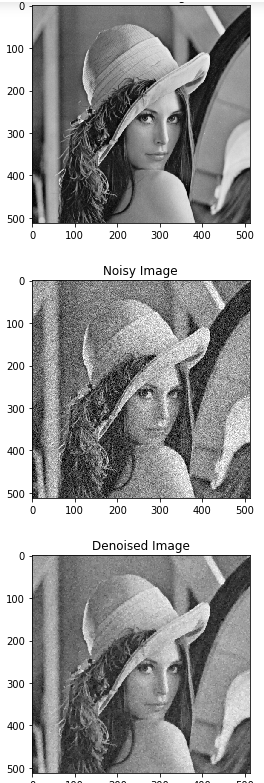• Introduction to supervised Machine Learning: A probabilistic introduction. PDF slides
• Connecting local models : The case of chains PDF slides
• Connecting local models : Beyond chains and trees.PDF slides
• The tutorial can be found here. HTML Notebook

### Transfer Learning for object classification with an integration to an Android application

Goals :
• Transfer learning: Fine tuning of MobileNet
• Integration an android application for custom object classification
• Speed comparison can be made according to computation device : CPU, GPU and NPU
The tutorial can be found here. PDF

### Reinforcement Learning by Q-Learning

Goals :
• An introduction to Q-Learning.
• An application to train a robot to escape a maze.
• Code in Python base Numpy and Matplotlib.
The tutorial can be found here. HTML Notebook

### The language of probability for machine learning: An application to skin detection thanks to the Naive bayes classifier

Goals :
• An introduction to probability and the bayes' rule.
• An introduction to fitting probability models: Maximum likelihood, Maximum a posteriori.
• An introduction to generative and discriminative models.
• Introduction to supervised Machine Learning: A probabilistic introduction. PDF slides
• Connecting local models : The case of chains PDF slides
• Connecting local models : Beyond chains and trees.PDF slides
The tutorial can be found here. HTML Notebook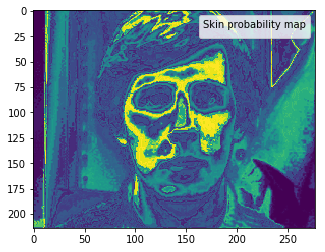### Simulation of self driving car

The material is there Code and Data
Simulation of Self Driving Car based on Thibault Neveu's tutorial (https://www.youtube.com/watch?v=JogUFFcfIYg&t=2118s). However, the prediction model has been adapted to fit the objective of an academic course. Especially, students have to develop from A to Z the learning algorithm (Stochastic Gradient Descent) and the prediction method (linear regression model)).
Why the least square errors ?? Slide Linear regression example on house prices

Gradient descent is an algorithm to find the parameters of a model. The gradient on its own can be a noisy. It means it changes a lot at each iteration. In order to get a clear trend on the gradient values, it is possible to make it smoother. This is where Momentum, RMSProp and ADAM appear. The Notebook is there

### Overfitting phenomenon of the maximum likelihood

A word about the variance (biased or not) The Notebook is there### Pedestrian image classification (in french)

When the cross entropy makes the problem convex !!!Slide
HTML version of the notebook can be found here. HTML Notebook

### Minimizing the cross entropy : a nice trip from Maximum likelihood to Kullback–Leibler divergence

In this notebook,
• We show that minimizing the cross entropy is equivalent to maximizing the likelihood in case of discriminative models.
• We show that minimizing the cross entropy is equivalent to minimizing the Kullback–Leibler divergence
• We show that the cross entropy loss makes the logistic regression problem convex
• We present an application to cats and dogs image classificationHTML version of the notebook can be found here. HTML Notebook

### Gaussian Mixture and Expectation Maximization algorithm

An illustration of fitting a data set with a Gaussian mixture The Notebook is there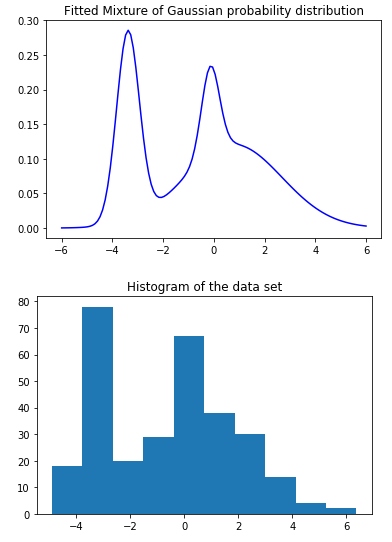### Minimizing the least squares error with quadratic regularization : but why

In this Notebook,
• we show that minimizing the least squares error in a regression context is related to a Gaussian assumption of the error distribution
• we also show that \$L2\$ regularization appears under an assumption of Gaussian distribution of the parameters and a Baysian treatment
• We show that maximizing the posterior distribution is equivalent to minimizing the regularized sum-of-squares error function with a quadratic regularizer
The Notebook is there

### Maximizing the margin : Support Vector Machines

Often in machine learning, we introducce a parametrized distribution and we search the parameters that maximizee the likelihood over some data. This can be equivalent to minimize an error function defined on the data (mean square error, mean cross entropy). In this notebook, we describe the Support Vector Machines (SVM) Problem. Previously we have seen that many loss functions in machine learning are related to Maximum Likelihood. See :
• Minimizing the cross entropy : a nice trip from Maximum likelihood to Kullback–Leibler divergence (http://romain.raveaux.free.fr/document/CrossEntropy.html)
• Overfitting phenomenon of the maximum likelihood (http://romain.raveaux.free.fr/document/Overfittingbiaisedandunbiaisedvariance.html)
• Gaussian Mixture and Expectation Maximization algorithm (http://romain.raveaux.free.fr/document/GaussianMixtureandExpectationMaximization.html)
• Relation between the least squares error the Maximum Likelihood (http://romain.raveaux.free.fr/document/LeastSquaresError.html)
The maximum likelihood can suffer from a severe over fitting phenomenon, for instance:
• A data set is not well fitted by Gaussian models (under estimation of the variance)
• In Gaussian mixture models, a Gaussian distribution can be fitted to a single data point
We have showed that the maximum likelihood can be equivalent to minimize an average error on a given data set (mean square error, mean cross entropy). But instead of minimizing a mean error, would it be more interesting to minimize the largest error? Would it offer a better generalization property.... This is where the idea of maximizing the margin comes from .... The Notebook is there

### Helicoptere simulation

Video from the press

### Graph in Pattern Recognition

Introduction PDF
Computer vision and Graph-based representation : PDF
Pattern recognition problems : PDF
Graph matching problems : PDF
Graph matching formulations : PDF
Graph matching methods : PDF
Graph embedding problems : PDF
Graph embedding methods : PDF
Booklet : PDF
Illustrations : PDF

Lecture : CM
TP1: TP1
TP2: TP2
TP3: TP3
TP4: TP4
TP5: TP5
TP6: TP6

Lecture : CM
TD: TD
TP : TP

### Android

Practical work (labs) on "Android Getting Started" is available : Download TP1. It presents different components of the SDK : DDMS, ADB, Eclipse ADT, ....

Android : Communication between Activities Practical work (labs) on passing and exchanging objects between activities are proposed. The main purpose is to understand how two different processes can communicate between them. Download TP2

Android : Local Services (Listener and Binder ) Practical work (labs) on Local Services are proposed. Services are tasks running in background. Such powerful concept lead us to the definition of Binders and Listeners. Binders are used to retrieve an instance of the running service while Listeners listen to data changes aiming at the GUI update. Download TP3

Android : Video in Android using C/C++ code Practical work (labs) on processing video frames using pure JAVA code and mixed C++/JAVA code are available. It aims at comparing application performances with and wihtout native code when dealing with video preview in Android. Download TP4 and Download TP5

Android : Content Provider Practical work (labs) on creating SQLLite databases and sharing data between applications by ContentProvider. Download TP6

Android : Read/Write XML files Practical work (labs) on parsing and writing XML files using DOM API. Download TD

Android : Treasure Hunt Practical work (labs) on realizing a full application : Treasure Hunt. Specifications DTD XML MAP

Canal_de_Marans.gpx

### Script Windows And Linux

Goals and Organization
Intro Script Linux Lecture
Appendix Script Linux
Script Windows Lecture
Appendix Script Windows
Android layers
Practical Work on Windows Script (TP1)
Practical Work on Windows Script (TP1 Extended)
Practical Work on Linux Script (TP2)

### Statistics

I led the talk about statistics. (Level: Master Degree 1st year). I created teaching material for that purposes.
• Courses can be found here : Principal Component Analysis (PCA) and Correspondence Factor Analysis (CFA). PCA CFA